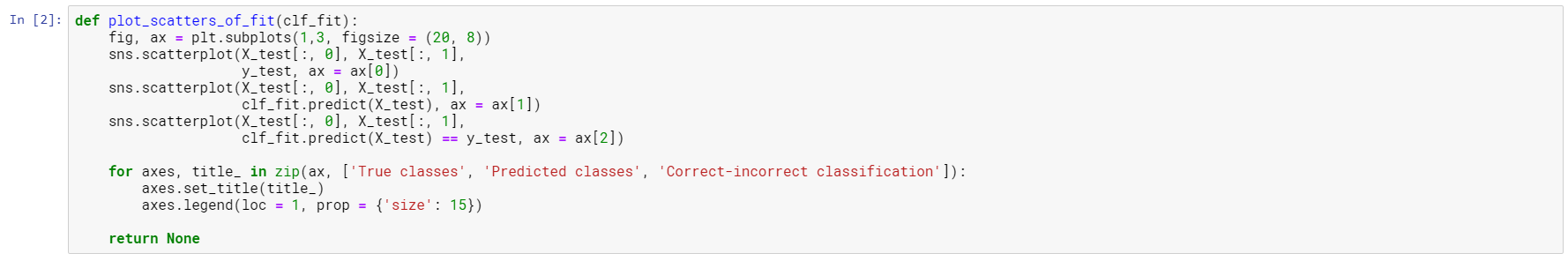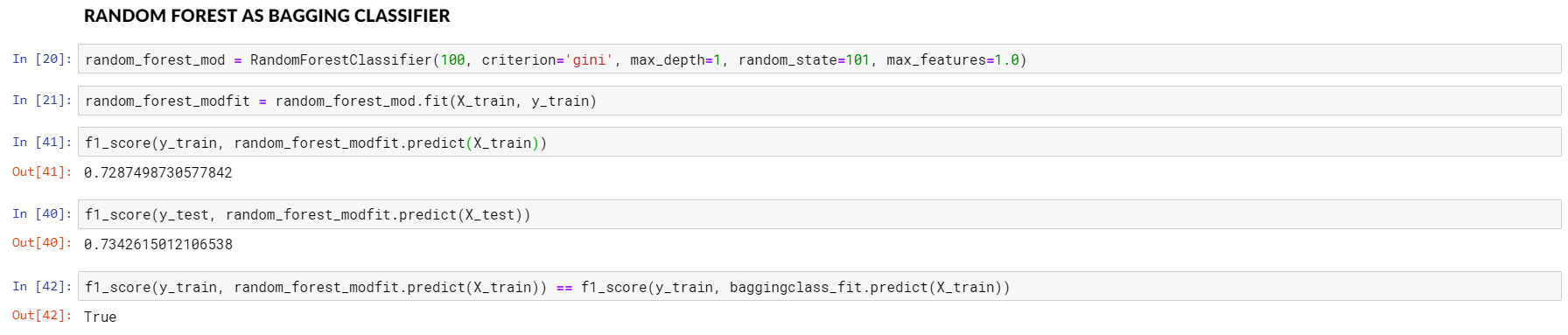# Digging deeper into ensemble learningSource: https://aperiodical.com/tag/statistics/

Have you ever wondered how combining weak predictors can yield a strong predictor? Ensemble Learning is the answer! This is the second of a pair of articles in which I will explore ensemble learning and bootstrapping, both the theoretical basics and real-life use cases. Let’s do this!

Part 1 of this blogpost about bootstrapping is available here!

You can look at the code on my GitHub:

#### Bagging: combining regression/classification trees

Now that we understood the principle underlying bootstrapping, let’s see how to make use of it an a machine learning context. Bagging stands for boostrap aggregating. This is a machine learning meta-algorithm for example Random Forest models make use of.

The bootstrapping part of bagging is done by re-sampling the training set n times, thereby creating n distinct training sets. Then, the same (preferably quite basic) machine learning model is fit on the training sets, predicting a target variable. Aggregation is then achieved by combining these models: in terms of classification, the fitted models ’vote’ for each class, and the class with the most votes gets predicted. In regression problems, the prediction is going to be the mean of the consisting models’ predictions.

Let’s show this on a hands-on example in Python! Making use of sklearn’s great make_circles functionality, we can create a quite difficult classification problem. Now let’s try to predict the classes of these points using their coordinates!

First, let’s try to solve our task with a simple decision tree. In order to avoid overfitting, the max_depth parameter is set to 1 — we only let the decision tree to perform a single cut. This is mainly done for didactic purposes, as this way it can be shown how combining many instances of an underfitting model can result in a pretty decently fitting model. The models are initialized with the same parameters for comparability. 25% of the data set is held back as a test set (the test set ratio), and for reproducibility, we set the random state to 42, for the obvious reasons.

So, let’s take a look at what a simple decision tree can achieve in this problem! We can see that it is rather underfitting. To evaluate classifier performance, f1_score is used. It is an equally weighted average of precision and recall, which gives a much better metric than the simple score of correct predictions ratio.

The three plots are going to show, throughout the article, the true classes, the predicted classes, and whether or not the prediction is correct. They are created using this function:Function to plot classes, predicted classes, and if a classification is correct or not.

Now let’s do some bagging! Fortunately, Python’s sklearn package has a class to perform bagging classification, called BaggingClassifier. Under the hood, it will create bootstrapped samples, and fit the same decision tree to each sample. The final predictions are then obtained by the fitted classifiers ’voting’ in each case in the way described above.

We can immediately see a significant improvement in the classification, both for the training and the test set. But why? In the first example of bootstrapping, we only got a distribution instead of a point estimate. Here we are actually better at out-of-sample prediction.

The answer is more of a practical one rather than one having a clear mathematical formulation. There is a related notion called Condorcet’s Jury Theorem. The theorem states that in a voting scheme, if an individual makes the right decision even just slightly more than 50% of the times, as more people vote the probability of the right outcome increases. As if making a decision, creating a prediction can be enhanced by adding together more predictors that are right at least 50% of the times.

The essence of this method is that as long as the predictors fitted on each bootstrapped sample are not perfectly correlated, the errors cancel each other out by averaging them. For a truly random pick of samples, the likelihood of a model overestimating the outcome is the same as for underestimating it, and this compensates for errors. (For a more detailed explanation, please consult Domingos (1997), or Section 8.7 in Hastie, Friedman and Tibshirani’s Elements of Statistical Learning (12th ed.). A detailed alternative explanation by Mike Liao is worth reading, too.)

Random Forest models are also making use of the bagging concept. Let’s try this, and see if we get the same results as with the BaggingClassifier!

It’s surprising, right? With seemingly the same model, we get better results just by repeating it. How can this be? The answer lies in the different default settings of these models, in particular the max_features parameter, which controls how many features each tree can consider to find the best split. If you check out the docs, you will see, that by default, Random Forest uses the square root number of features for each model built on bootstrapped samples, while Bagging Classifier uses all.

Consequently, models built using the Random Forest algorithm are less correlated, therefore less likely to make the same mistake — making it more likely that errors cancel out each other through the agregation process. You can easily validate this by specifying the max_features parameter of Random Forest.Random Forest Classifier is just a Bagging Classifier after all…

#### Building trees more cleverly: boosting

A more recent advancement in ensemble learning is the concept of boosting. In using bagging, we built trees simultaneously on bootstrapped samples. Boosting methods, on the other hand, such as Gradient Boosting Trees, Adaboost and XGBoost, build trees sequentially. And in every iteration, the performance of trees gets better and better. The algorithm works as follows.

1. A first tree is built, then evaluated.

2. Upon building the next tree, extra weight is given to misclassified observations — effectively ’guiding’ the algorithm to pay more attention to these data points. This is done through the class_weights argument of the model, which controls what weight is attributed to each observation in the loss function.

3. This process goes on until a predefined number of trees are built. This way, the models are even less correlated, and additional weight is given to hard-to-classify observations.

The results speak for themselves:

#### Takeaways

After getting to know bootstrapping in Part 1, we saw in this Part 2 how bagging and boosting make use of this simple concept. We learned about how the uncorrelated errors of weak predictors cancel out each other for better out-of-sample fit, and that in some cases, fewer features yield a better overall outcome — as we saw in the case of Random Forest. In addition, we have seen that an algorithm that prioritises and weights incorrectly predicted cases can also help developing a better model. These steps helped us raise our test score from 57% to 90% in this particular classification case. And you, too, can make use of these techniques to make the best of your data by leveraging an ensemble of multiple predictors to achieve better overall performance.